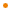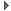# JavaFX: Bringing Rich Experiences To All the Screens Of Your Life

### Overview

`DateTime` represents a specific instant in time, with millisecond precision. Unlike `java.util.Date`, `DateTime` supports the "proleptic Gregorian calendar" (the pure Gregorian calendar with no Julian-Gregorian transition).

Profile: common

### Variable Summary

accessnametypedescription
public-initinstantLongthe instant at which this DateTime was instantiated

The instant value represented by the number of milliseconds since the Epoch (1970-01-01T00:00:00Z).

### Script Function Summary

public impl_parseRFC822DateTime(input: java.lang.String) : DateTime

Parses the given `input` in the RFC 822 date-time format and returns a `DateTime` object.Parses the given `input` in the RFC 822 date-time format and returns a `DateTime` object.

Any military single letter zone is converted to its corresponding custom time zone name, such as "A" converted to "GMT-01:00".

impl_toRFC822String()

Parameters
input
the string in the RFC 822 date-time format to be parsed
Returns
DateTime
Throws
&nbsp;
public impl_parseXMLDateTime(input: java.lang.String) : DateTime

Parses the given input in an ISO 8601 format and returns a `DateTime` object.Parses the given input in an ISO 8601 format and returns a `DateTime` object.

Parameters
input
Returns
DateTime
&nbsp;

### Function Summary

public impl_toRFC822String() : java.lang.String

Converts this `DateTime` object to a `String` of the form defined by RFC 822:Converts this `DateTime` object to a `String` of the form defined by RFC 822:

``````
EEE ', ' dd ' ' MMM ' ' yyyy ' ' HH ':' mm ':' ss ' ' Z
``````
where
• EEE is a day of week abbreviation, such as "Sun".
• dd is a two-digit number representing day of month (01 - 31).
• MMM is a month abbreviation, such as "Jan".
• yyyy is a number representing the year.
• HH is a two-digit number representing the hour in the 24-hour clock (00 - 23).
• mm is a two-digit number representing the minute (00 - 59).
• ss is a two-digit number representing the second (00 - 59).
• Z is either a zone name defined by RFC 822 or sign followed by 4-digit time zone offset, such as "-0800". No military single letter zone representation is supported for formatting.
• ', ', ' ', ':' are separaters between fields.

Returns
String
&nbsp;
public impl_toString() : java.lang.String

Converts this `DateTime` object to a `String` of the form:Converts this `DateTime` object to a `String` of the form:

```   ['-']yyyy '-' MM '-' dd 'T' hh ':' mm ':' ss['.' f] [zzzzzz]
```
where
• [ '-' ] is an optional negative sign indicating the year is before 1. (The year before 1 is represented as "-0001".)
• yyyy is a four-or-more digit number representing the year.
• '-' is a date field separater.
• MM is a two-digit number representing the month (01 - 12).
• dd is a two-digit number representing the day of month (01 - 31).
• 'T' is a separater between the date fields and time fields.
• hh is a two-digit number representing the hour in the 24-hour clock (00 - 23).
• ':' is a time field separater.
• mm is a two-digit number representing the minute [00-59].
• ss is a two-digit number representing the second (00 - 59).
• ['.' f] is an optional number representing the fractional seconds. For example, if the fractional seconds value is 120 milliseconds, it is represented as ".12". If the value is 0, this part is empty.
• [zzzzzz] represents optional time zone information as 'Z' | sign hh ':' mm, where:
• 'Z' represents UTC.
• sign is character `'+'` representing a positive time zone offset, or character `'-'` representing a negative time zone offset.
• hh is a two-digit number representing hours (00 - 14).
• ':' is a separater between hours and minutes.
• mm is a two-digit number representing minutes (00 - 59).
If this `DateTime` object has no time zone, this part is empty.

Returns
String
&nbsp;

### Inherited Functions

Spec-Zone.ru - all specs in one place